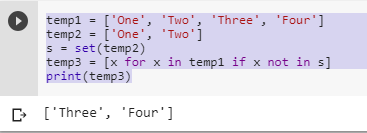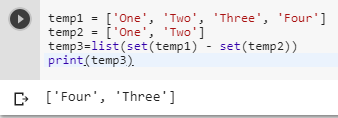3 views
in Python

I have two lists in Python, like these:

temp1 = ['One', 'Two', 'Three', 'Four']

temp2 = ['One', 'Two']

I need to create a third list with items from the first list which aren't present in the second one. From the example I have to get:

temp3 = ['Three', 'Four']

Are there any fast ways without cycles and checking?

by (106k points)
• There are many ways of getting unique values from the above two lists some of them are as follows:-

• The first thing we can do is use list comprehension.

s = set(temp2)

temp3 = [x for x in temp1 if x not in s]

• Full code of this is:-

temp1 = ['One', 'Two', 'Three', 'Four']

temp2 = ['One', 'Two']

s = set(temp2)

temp3 = [x for x in temp1 if x not in s]

print(temp3)• Another way we can solve the above problem by using list(set(temp1) - set(temp2)) method.

• Code of this is:-

temp1 = ['One', 'Two', 'Three', 'Four']

temp2 = ['One', 'Two']

temp3=list(set(temp1) - set(temp2))

print(temp3)